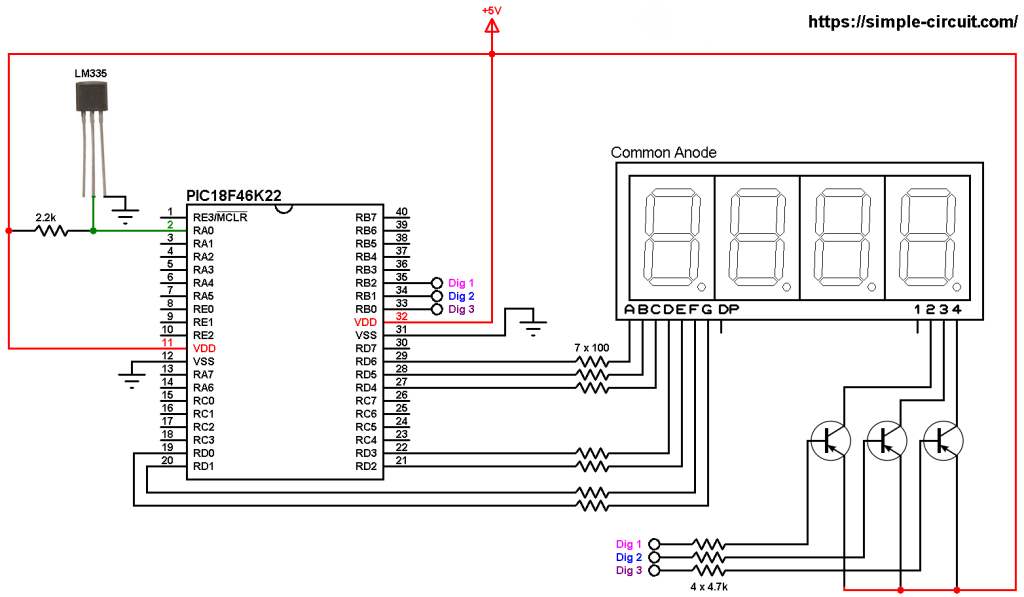# Interfacing PIC18F46K22 with LM335 sensor and 7-segment display

This PIC18F46K22 microcontroller project shows how to read temperature from LM335 analog temperature sensor and print its value on a three-digit seven-segment display.
The LM335 sensor is a 3-pin analog device which can measure temperature (converts temperature to analog voltage). This sensor requires an ADC module to convert the analog voltage into digital data. The PIC18F46K22 microcontroller has one ADC module with 10-bit resolution.
ADC: Analog-to-Digital Converter.

The LM335 sensor has the following features (from datasheet):

• Directly Calibrated to the Kelvin Temperature Scale
• 1°C Initial Accuracy Available
• Operates from 400 μA to 5 mA
• Less than 1-Ω Dynamic Impedance
• Easily Calibrated
• Wide Operating Temperature Range
• 200°C Overrange
• Low Cost

The LM335 has a breakdown voltage directly proportional to absolute temperature at 10 mV/°K. For example if the LM335 output voltage is equal to 3.03 (3030 mV) this means the temperature is: 303 Kelvin = 30 °Celsius.

The PIC18F46K22 is an 8-bit microcontroller which has 10-bit ADC module. The good thing with this microcontroller is the fixed voltage references. With the fixed voltage reference we get a very good result. Normally negative and positive references of the ADC module are VSS and VDD, but VDD is not exactly equal to 5.00V and here we should use the fixed voltage reference as a positive reference of the ADC module.

The PIC18F46K22 has 3 fixed voltage references: 1.024V, 2.048V and 4.096V. For example if we set the fixed voltage reference to 4.096V and the ADC module is configured so that the negative and the positive references are VSS and FVR (Fixed Voltage Reference) respectively, in this case the equivalent 10-bit digital value of 4.096 is 1023 and 3.00V is 3.00 * 1023/4.096 = 749 , and so on.
In this project I used 4.096 because the LM335 output is between 2.23V (temperature = -50°C) and 3.98V (temperature = +125°C).

The value of the temperature is displayed on 3-digit common anode 7-segment display, to see how to interface the PIC18F46K22 microcontroller with 7-segment display (4-digit counter example), visit the following post:
Interfacing PIC18F46K22 with 7-segment display | 4-Digit counter example

Parts Required:

• PIC18F46K22 microcontroller   —->  datasheet
• 3 or 4-digit common anode 7-segment display
• LM335 temperature sensor   —->   datasheet
• 3 x PNP transistor (2SA1015, 2S9015, 2N3906 …)
• 7 x 100 ohm resistor
• 3 x 4.7k ohm resistor
• 2.2k ohm resistor
• 5V source
• Breadboard
• Jumper wires

PIC18F46K22 with LM335 sensor and 7-segment display circuit:
Project circuit schematic diagram is shown below.All the grounded terminals are connected together.

The LM335 sensor has 3 pins (from left to right):
Pin 1 for calibration, not used in this example.
Pin 2: output.
Pin 3: GND (ground).

The output pin of the LM335 sensor is connected to pin RA0 which is analog channel 0 (AN0). I chose the 2.2k ohm because as written in the datasheet for optimum accuracy the current flows through the LM335 should be 1mA. For example if the temperature = 27°C, the output will be 3.00V and assume the supply voltage is exactly 5.00V that means the current flows through the sensor is ( 5 – 3)/2.2 = 0.90mA which is good enough. Also the value 2.2k is a standard value and well used.

The 3 transistors are of the same type (PNP).

In this project the PIC18F46K22 microcontroller runs with its internal oscillator @ 8 MHz, MCLR pin is configured as an input pin.

PIC18F46K22 with LM335 sensor and 7-segment display C code:
The following C code is for CCS C compiler, it was tested with version 5.051.

The internal fixed voltage reference (FVR) of the PIC18F46K22 microcontroller is set to 4.096V using the following line:

The ADC module is configured so that it uses its internal clock, voltage references (negative and positive) are set to VSS and FVR respectively where the FVR is the one previously set to 4.096V.
The LM335 output pin is connected to AN0 channel. All these configurations are done in the code as shown below:

PIC18F46K22 ADC module is used with 10-bit resolution which means the digital value of the input analog voltage varies between 0 (0V) and 1023 (4.096V). By multiplying the digital value by 0.4 we get the temperature in Kelvin (0.4 = 100*4.096/1024). The temperature in degree Celsius = Kelvin – 273.

CCS C Code:

Proteus simulation of the project should give a result near to the one shown below where Arduino UNO board is used (simulation circuit is not the same as real hardware circuit, project hardware circuit is shown above):

This site uses Akismet to reduce spam. Learn how your comment data is processed.